# 2nd PUC Economics Model Question Paper 3 with Answers

Students can Download 2nd PUC Economics Model Question Paper 3 with Answers, Karnataka 2nd PUC Economics Model Question Papers with Answers helps you to revise the complete Karnataka State Board Syllabus and score more marks in your examinations.

## Karnataka 2nd PUC Economics Model Question Paper 3 with Answers

Time: 3 hrs 15 minutes
Max. Marks: 100

PART-A

I. Choose the correct answer (1 × 5 = 5)

Question 1.
Which of the following is an example of microeconomic study?
(a) National income
(b) Consumer behaviour
(c) Unemployment
(d) Foreign trade
Answer:
(b) Consumer behaviour

Question 2.
The formula of production function is
(a) q=f(L,K)
(b) q=d(p)
(c) Y=f(x)
(d) None of the above
Answer:
(a) q = f(L,K)

Question 3.
Profit =
(a) PxQ
(b) TR-TC
(c) TFC + TVC
(d) TR/Q
Answer:
(b) TR-TC

Question 4.
Measuring the sum total of all factor payments will be called
(a) Product method
(b) Expenditure method
(c) Income method
(d) None of the above
Answer:
(c) Income method.Question 5.
The banks which are a part of the money cheating system of the economy are
(a) Bankers
(b) Commercial banks
(c) RBI
(d) None of the above
Answer:
(b) Commercial banks

II. Fill in the blanks. (1 × 5 = 5)

Question 6.
In a centrally planned economy, all important decisions are made by_____
Answer:
Government.

Question 7.
In monopoly market, the goods which are sold have no______
Answer:
Substitutes.

Question 8.
_____will be called as firms.
Answer:
Production units.

Question 9.
_____is the only institution which can issue currency in India.
Answer:
RBI.Question 10.
_____is defined as addition to the stock of physical capital.
Answer:
Investment

III. Match the following. (1 × 5 = 5)

Question 11.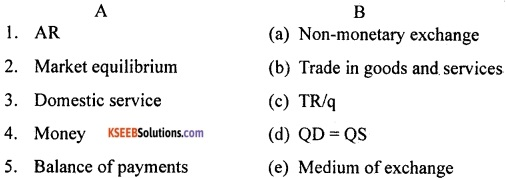Answer:
1-c; 2-d; 3-a; 4-e; 5-b.

IV. Answer the following questions in a sentence or a word each. (1 × 5 = 5)

Question 12.
What is normal profit?
Answer:
The minimum level of profit that is needed to keep a firm in the existing business is called as normal profit.

Question 13.
What is price floor?
Answer:
The government imposed lower limit on the price that may be charged for a particular good or service is called price floor. Example, agricultural price support programmes and minimum wage legislation.

Question 14.
What is duopoly?
Answer:
It is a special case of oligopoly where there are exactly two sellers or firms.Question 15.
Who are economic agents?
Answer:
Economic agents are those individuals or institutions which take economic decisions. They can be consumers, producers, government, corporation, banks, etc.

Question 16.
Give the meaning of intermediate goods.
Answer:
These are the goods used by other producers as material inputs. These are used as raw material for production of other commodities. These are not final goods.

PART-B

V. Answer any NINE of the following in 4 sentences each. (2 × 9 = 18)

Question 17.
What is monotonic preference?
Answer:
A consumer’s preferences are said to monotonic if and only if between any two bundles, the consumer prefers the bundle which has more of at least one of the goods and no less of the other good as compared to the other bundle.

For instance, the consumer between any bundles say (x1, x2) and (y1, y2), if (x1, x2) has more of at least one of the goods and no less of the other good compared to (y1, y2) then the consumer prefers (x1, x2) to (y1, y2). This is called monotonic preferences.

Here, the consumer will not remain indifferent between two combinations of commodities when he has an opportunity to have more quantity in one combination than the other.

Question 18.
What do you mean by price elasticity of demand?
Answer:
Price elasticity of demand is a measure of the responsiveness of the demand for a good to change in its price. In the words of Prof. Stonier & Hague, “Price elasticity of demand is a technical term used by economists to describe the degree of responsiveness of the demand for a good to a change in its price. It is measured by using the following formula.
$$\mathrm{PED}=\frac{\text { Percentage chánge in demand for the good }}{\text { Percentage change in price of the good }}$$Question 19.
Mention the types of returns to scale.
Answer:
The types of returns to scale are:

1. Constant returns to scale.
2. Increasing returns to scale.
3. Decreasing returns to scale.

Question 20.
Write the meaning of opportunity cost with an example.
Answer:
Opportunity cost of some activity is the gain foregone from the second-best alternative activity. For example, you have Rs. 10000 which you decide to invest in your family business. What is the opportunity cost of your action? If you do not invest this money, you can either keep it in the house safe which will give you zero return or you can deposit it in either bank A or bank B in which case you get an rest at the rate of 20% or 10%, respectively.

So the maximum benefit that you may get from other alternative activities is the interest from the bank A. But this Opportunity will no longer be there once you invest the money in your family business. The opportunity cost of investing the money in your family business is, therefore, the amount of forgone interest from the bank A.

Question 21.
How wage is determined in the labour market?
Answer:
The wage rate is determined at the point where the labour demand and supply curves intersect. This is shown in the following diagram: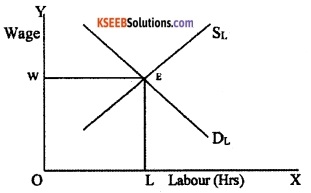In the above diagram, hours of labour is measured in X-axis and Wage is measured in Y-axis. SL is labour supply curve and DL is labour demand curve. With an upward-sloping supply curve and downward sloping demand curve, the equilibrium wage rate is determined at the point where these two curves intersect (point E). That means the wage rate is determined at that point where the labour which the households wish to supply is equal to the labour that the firms wish to hire.

Question 22.
Mention the requirements of a monopoly market structure.
Answer:
The requirements of a monopoly market structure are as follows:

1. Existence of a single producer of a particular commodity.
2. No other commodity works as a substitute for this commodity.
3. The monopoly situation has to persist over a time.
4. Restrictions to prevent other firms entering the market and selling the commodity.

Question 23.
What is the difference between consumer goods and capital goods?
Answer:

 Consumer Goods Capital Goods • These are the goods which are purchased for consumption by ultimate consumers. • Examples are food, clothes and service like recreation. • These are the durable goods which are used in the production process. • Examples are machinery, tools, implements, etc.

Question 24.
Mention three methods of measuring GDP (national income).
Answer:
The three methods of measuring GDP are:

1. Product or value added method.
2. Expenditure method.
3. Income method.Question 25.
Give the meaning of paradox of thrift.
Answer:
As people become more thrifty, they end up saving less or same as before in aggregate, known as paradox of thrift. In other words, if all the people of the economy increase the proportion of income they save, total value of savings in the economy will not increase it will either decrease or remain unchanged. This result is known as the paradox of thrift.

Question 26.
What are the factors which cause change in aggregate demand?
Answer:
The factors which cause change in aggregate demand are as follows:

• Change in consumption.
• Change in investment.

Question 27.
Mention the non-tax revenues of the central government.
Answer:
The non-tax revenues of the central government mainly consists of the following:

1. Interest receipts on account of loans by the central government.
2. Dividends and profits on investments made by the government.
3. Fees and other receipts for services rendered by the government.
4. Grants-in-aid from foreign countries and international organizations.

Question 28.
Why the proportional income tax acts as automatic stabilizer?
Answer:
The proportional income tax acts as an automatic stabilizer because it makes disposable income and consumer spending less sensitive to fluctuations in GDP. When GDP rises, disposable income also i rises but by less than the rise in GDP because a part of it is siphoned off as taxes. This helps to limit the upward fluctuation in consumption spending.

During a recession when GDP falls, disposable income falls less sharply and consumption does not drop as much as it otherwise would have fallen, had the tax liability been fixed. This reduces the fall in aggregate demand and stabilizes the economy.

Question 29.
Why do people demand foreign exchange?
Answer:
People demand foreign exchange rate because of the following reasons:

1. To purchase goods and services from other countries.
2. To send gifts abroad.
3. To purchase financial assets abroad.

Question 30.
Differentiate between depreciation and devaluation.
Answer:
The difference between depreciation and devaluation is as follows:

 Depreciation Devaluation • Here, the price of foreign currency in terms of domestic currency increases. • Here, the government deliberately makes the domestic currency cheaper by increasing exchange rate. • It happens because of market forces, i.e. demand for foreign exchange and supply of foreign exchange. • It is a deliberate action of government.

PART-C

VI. Answer any SEVEN of the following questions in 12 sentences each. (4 × 7 = 28)

Question 31.
Briefly explain the central problems of an economy.
Answer:
An economic system or economy is a mechanism where the scarce resources are channelized on priority to produce goods and services. These goods and services produced by all the sectors of the economy determine the national income.

Generally, human wants are unlimited and resources to satisfy them are limited. If there was a perfect match between human wants and availability of resources there would have been no scarcity, no problem of choice and no economic problems at all. So, one has to select the most essential want to be satisfied with limited resources. In economics, this problem is called ‘problem of choice’.

The problem of choice arising out of limited resourced and unlimited wants is called economic problem. Every economy whether developed or underdeveloped, capitalistic or socialistic or mixed economy, there will be three basic economic problems, viz. what to produce? how to produce? and for whom to produce? Let us discuss in detail.

a. What to produce, i.e. what is to be produced and in what quantities?
Every country has to decide, which goods are to be produced and in what quantities. Whether more guns should be produced or more food grains should be grown or whether more capital goods like machines, tools, etc. should be produced or more consumer goods (electrical goods, daily usable products, etc.) will be produced.

What goods to be produced and in what quantity depends on the economic system of the country. In socialistic economy, the government decides and in capitalistic economy market forces decides and in mixed economy both the government and market forces provide solutions to this problem.

b. How to produce, i.e. how are goods produced?
There are various alternative techniques of producing a product. For example, cotton cloth can be produced with either handloom or power looms. Production of cloth with handloom requires more labour and production with power loom use of more machines and capital. It involves selection of technology to produce goods and services.

There are two types of techniques of production, viz. labour-intensive technology and capital intensive technology. The society has to decide whether production be based on labour-intensive or capital intensive techniques. Obviously, the choice of technology would depend on the availability of different factors of production (land, labour, capital) and their relative prices (rent, wages, interest).

c. For whom to produce, i.e. for whom are the goods to be produced?
Another important decision which an economy has to take is for whom to produce. The economy cannot satisfy all wants of all the people. Therefore, it has to decide who should get how much of the total output of goods and services. The society has to decide about the shares of different groups of people poor, middle class and the rich, in the national output.

Thus, every economy faces the problem of allocating the scarce resources to the production of different possible goods and services and of distributing the produced goods and services among the individuals within the economy. The allocation of scarce resources and the distribution of the final goods and services are the central problems of any economy.Question 32.
Write the differences between total utility and marginal utility.
Answer:

 Total Utility Marginal Utility • It is the aggregate utility derived by the consumer by consuming all the units. • It is the additional utility derived by the consumer by consuming additional unit. • It represents utility of all the units consumed. • It represents the utility of single unit. • It may be symbolically written as. TUn=U1+U2+U3+U4………….. Un. • It may be written as MUn = TUn – TUn-1 • It increases in the beginning and later decreases as the consumer consumes more and more units. • It decreases from the beginning and becomes negative later.

Question 33.
Write the differences between substitutes and complements.
Answer:

 Substitute Goods Complementary Goods • These are alternative goods available to satisfy our wants. • These are the goods which are consumed together. • If the price of a product increases, the demand for its substitute also increases. • If the price of a product increases, the demand for its complementary good decreases. • Examples for substitute goods are tea and. coffee, Colgate and Pepsodant, etc. • Examples for complementary goods are pen and ink, shoes and socks, etc. • Here the demand curve shifts to the right in case of price rise. • Here the demand curve shifts to left in case of price rise. • Price and demand move in same direction. • Price and demand move in opposite directions.

Question 34.
Explain the differences between normal and inferior goods with examples
Answer:

 Normal Goods Inferior Goods • These are the goods for which the demand increases with the increase in the income of consumer. • These are the goods for which the de­mand decreases with the increase in the income of consumer. • Examples for normal goods are food, cloths, electronic goods, luxury goods, etc. • Examples for inferior goods are low qual­ity of goods like unbranded products. • There is positive relationship between in­come and demand. • There is inverse relationship between income and demand. • Here the demand curve shifts towards right, if the income of consumer increases. • Here the demand curve shifts towards left, if the income of consumer increases.

Question 35.
Explain TP, MP and AP with the example.
Answer:
The TP is total product, MP is marginal product and AP is average product.
1.Total product:
Total product is the relationship between a variable input and output when all other inputs are held constant. Suppose we vary a single input and keep all other inputs constant. Then for different levels of that input, we get different levels of output. This relationship between the variable input and output, keeping all other inputs constant, is often referred to as total product of the variable input.

2. Average product:
Average product is defined as the output per unit of variable input. We calculate it as APL=TPL/L, where APL is the average product of labour, TPL is the total product of labour and L is the amount of labour input used.

3. Marginal product:
Marginal product of an input is defined as the change in output per unit of change in the input when all other inputs are held constant. It is the additional unit of output per additional unit of variable input. It is calculated by dividing the change in output by change in input labour.
MPL = ∆TPL/∆L
The concepts of TP, AP and MP can be explained with the help of following table: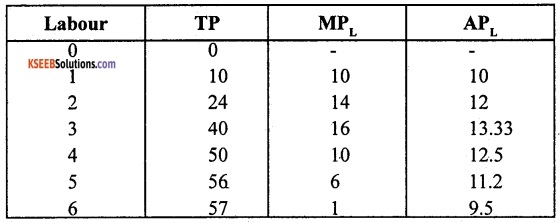The above table shows the total product of labour, marginal product of labour and average product of labour. The total product is also sometimes called as total return to or total physical product of the variable input labour. The third column gives us a numerical example of marginal product of labour.

The values in this column are obtained by dividing change in TP by change in labour. The last column gives us a numerical example of average product of labour. The values in their column are obtained by dividing TP by labour.

Question 36.
Explain the long-run costs.
Answer:
In the long run, all inputs are variable. There are no fixed costs, The total cost and the total variable cost coincide in the long run. There are two types of long-run costs. They are as follows:

a. Long-run average cost (LRAC):
The long-run average cost is the cost per unit of output produced. It is obtained by dividing the total cost by the output produced. It can be calculated as follows:
LRAC = TC/q
Where, TC is total cost and ‘q’ is quantity of output produced.

b. Long-run marginal cost:
The long-run marginal cost is the change in total cost per unit of change in output. When output changes in discrete units, then, if we increase production from q1 – 1 to q1 units of output, the marginal cost of producing q1th unit will be measured as follows:
LRMC = (TC at q 1 units) – (TC at q1 -1 units) or LRMC = TCn – TCn-1.Question 37.
Explain the average revenue or price line of a firm under perfect competition with the help of a diagram.
Answer:
1. Average revenue:
It refers to the revenue per unit of output sold. It is obtained by dividing the total revenue by the number of units of output sold. The average revenue is defined as total revenue per unit of output.
So, $$\mathrm{AR}=\mathrm{TR} / \mathrm{Q}=\frac{\mathrm{p} \times \mathrm{q}}{\mathrm{q}}=\mathrm{p}$$

Where, AR is average revenue, TR is total revenue y and Q is quantities sold. That means, for a price-taking firm, average revenue equals the market price price.

Under perfect competition, the AR will be equal to the market price. This is because, in perfect competitive market, the seller sells his product at the same price which is prevailing in the market. If the seller sells at low price, he incurs losses or if he increases the price, he loses customers. This can be represented in diagram: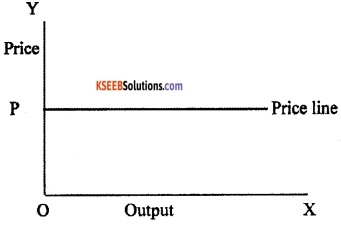In the above diagram, the average revenue for different values of firm’s output is shown in Y and X-axis respectively. Since the market price is fixed at P, we obtain a horizontal straight line that cuts the Y-axis at a height equal to P. This horizontal straight line is called the price line.

It is also firm’s AR curve under perfect competition. The AR curve of a firm is also the demand curve of the customers because the price paid by the consumer for each unit is the average revenue from the seller’s point of view.

Question 38.
Explain the working of the economy of a capitalist country.
Answer:
Capitalist economy can be defined as an economy in which most of the economic activities have the following characteristics:

1. There is private ownership of means of production.
2. Production takes place for selling the output.
3. There is sale and purchase of labour service at a price called wage rate.

In a capitalist country, production activities are mainly carried out by capitalist enterprises. A typical capitalist enterprise has one or several entrepreneurs. Entrepreneurs are those who exercise control over major decisions and bear a large part of the risk associated with the firm. They may themselves supply the capital needed to run the enterprise or they may borrow the capital.

To carry out the production they also need natural resources. They need the most important element of human labour to carry out production. This is called as labour. After producing output with the help of land, labour and capital, the entrepreneur sells the product in the market to earn money called revenue. Part of the revenue is paid out as rent for land, interest for capital and wage for labour and keeps the rest of the revenue as profit.

Profits are often used by the producers in the next period to buy new machinery or to build new factories, so that production can be expanded. These expenses which raise productive capacity are examples of investment expenditure.

Question 39.
Write a short note on the concept of final good.
Answer:
The final goods are those goods which are meant for final use and will not pass through any more stages of production or transformations. They are called final goods because once they have been sold they pass out of the active economic flow. However, they may undergo transformation by the action of the ultimate purchaser.

In fact, many final goods are transformed during their consumption. For instance, tea leaves purchased by the consumer are not consumed in that form – they are used to make drinkable tea, which is consumed. Similarly most of the items that enter our kitchen are transformed through the process of cooking.

But cooking at home is not an economic activity, even though the product involved undergoes transformation. Home-cooked food is not sold to the market. However, if the same cooking or tea was done in hotel where the cooked product would be sold to customers, then the same items are not considered as final goods and would be counted as inputs to which economic value addition can take place.

Thus, it is not in the nature of the good but in the economic nature of its usage that a good becomes a final good.

Question 40.
Explain the circular flow of income of an economy.
Answer:
The circular flow of income of an economy can be explained with the help of following assumptions:

1. Existence of two sectors, viz. household sector and producers.
2. Households are the owners of the factors of production.
3. Households receive income by selling the factor services.
4. There are no savings.
5. The firms produce goods to the households.
6. The economy is a closed economic system (where no government or external trade or savings).

The circular flow of income in a simple economy can be illustrated with the help, of following chart.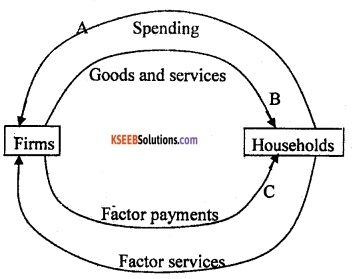In the above chart, the uppermost arrow, going from the households to the firms, represents the spending by the households to buy goods and services produced by the firms. The second arrow going from the firms to the households is the counterpart of the arrow above. It stands for the goods and services, which are flowing from the firms to the households.

Thus, the two arrows on the top represent the goods and services market the arrow above represents the flow of payments for the goods and services, the arrow below represents the flow of goods and services. The two arrows at the bottom of the diagram similarly represent the factors of the production market.

The lowermost arrow going from the households to the firms symbolizes the services that the households re-rendering to the firms. Using these services, the firms are producing the output. The arrow above this, going from the firms to the households, represents the payments made by the firms to the households for the services provided by the households.

Thus, when the income is spent on the goods and services produced by the firms, it takes the form of aggregate expenditure received by the firms. Since the value of expenditure must be equal to the value of goods and services, we can measure the aggregate income by calculating the aggregate value of goods and services produced by the firms. This is clearly shown above in the form of circular flow of income.Question 41.
Briefly explain the functions of money.
Answer:
The functions of money are as follows:

1. Medium of exchange:
Money plays an important role as a medium of exchange. It facilitates exchange of goods for money. It has solved the problems of barter system. Money has widened the scope of market transactions. Money has become a circulating material between buyers and sellers.

2. Measure of value/unit of account:
The money acts as a common measure of value. The values of all goods and services can be expressed in terms of money.

3. Stork of value:
People can save part of their present income and hold the same for future. Money can be stored for precautionary motives needed to overcome financial stringencies. Money solves one of the deficiencies of barter system, i.e. difficulty to carry forward one’s wealth under the barter system.

4. Transfer of value:
Money acts as a transfer of value from person to person and from place to place. As a transfer of value, money helps us to buy goods, properties or anything from any part of the country or the world. Further, money earned in different places can be brought or transferred to anywhere in the world.

PART-D

VII. Answer any Six of the following questions in 20 sentences each. (6 × 4=24)

Question 42.
Explain the consumption and investment function with the help of graphs.
Answer:
In a two-sector model, there are two sources of final demand. The first is consumption and the second is investment. The investment function was shown as I = I.
Graphically, this is shown as a horizontal line at a height equal to I above the horizontal axis.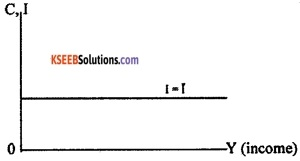In this model, I is autonomous which means, it is the same no matter whatever is the level of income. The consumers demand can be expressed by the equation C = $$\hat{\mathrm{C}}$$ + cY, where $$\hat{\mathrm{C}}$$ is autonomous expenditure and c is the marginal propensity to consume.
The consumption function can be graphically expressed as follows: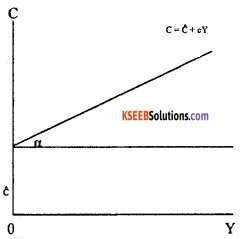In the above diagram $$\hat{\mathrm{C}}$$ is the intercept of the consumption, ‘c’ is slope of consumption function equals α.

Question 43.
Write the chart of components of current account.
Answer:
The chart which consists of different components of current account can be drawn as follows: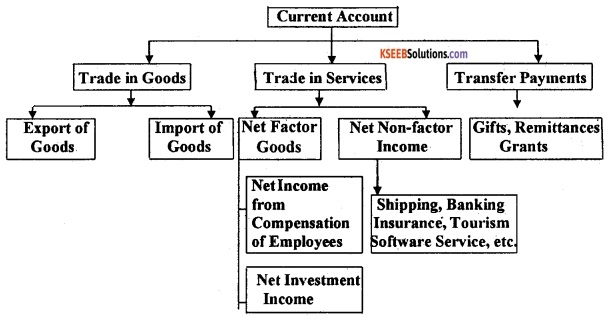Question 44.
Briefly explain the effect of an increase in demand for imports in the foreign exchange market with the help of a diagram.
Answer:
Foreign exchange market is the market in which national currencies are traded for one another. The major participants in the foreign exchange market are commercial banks, foreign exchange brokers and other authorized dealers and monetary authorities.

Foreign exchange rate is the price of one currency in terms of another currency. Different countries have different methods of determining their currency’s exchange rate. It can be determined through flexible exchange rate, fixed exchange rate or managed floating exchange rate.

The flexible exchange rate is determined by the market forces of demand and supply. Here, the exchange rate is determined at that point where the demand curve intersects with the supply curve.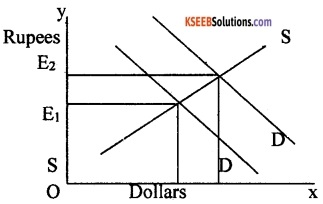If the demand for foreign goods and services increases; the demand curve shifts upward and right to the original demand curve. This can be graphically represented as follows:

The increase in demand for imports results in a change in the exchange rate. The initial exchange rate is E1 = 60, which means that we need to exchange Rs.60 for one dollar. At the new equilibrium, the exchange rate becomes E2 = 70, which means that we need to pay more rupees for a dollar.

The increase in the price of dollars due to rise in demand for imports indicates that the value of rupees in terms of dollars has fallen and the value of dollar in terms of rupees has increased.Question 45.
Explain the features of indifference curves with the help of diagrams.
Answer:
The main features of indifference curves are as follows:

1. Indifference curve slopes downwards from left to right:
An indifference curve slopes towards from left to right because, the consumer in order to have more of one product, he has to forego some units of other product. This can be explained with the help of diagram.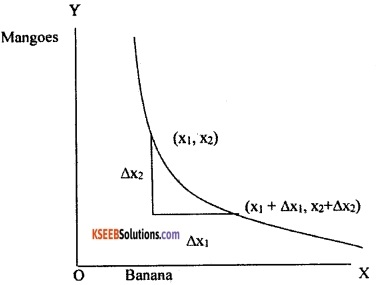Thus, according to above diagram, as long as the consumer is on the same indifference curve, an increase in bananas must be compensated by a fall in quantity of mangoes. That means an increase in the amount of bananas along the indifference curve is associated with a decrease in the amount of mangoes.

2. Higher indifference curve gives greater level of utility:
As long as marginal utility of a commodity is positive, a consumer always prefers more of that commodity to increase his level of satisfaction. This can be explained with the help of table and a diagram:

 Combination Banana Mango A 1 10 B 2 10 C 3 10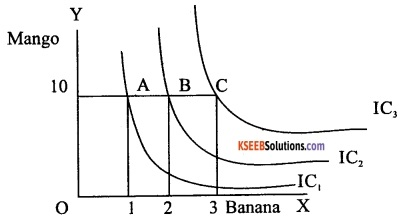Let us consider the different combinations of two goods bananas and mangoes A, B and C in the above table and diagram. All the three combinations consist of same quantity of mangoes but different quantities of bananas. As combination B has more bananas than A, B will provide the consumer higher level of satisfaction than A.

Therefore, B will lie on higher indifference curve. Similarly, C has more bananas than B and therefore C will provide higher level of satisfaction than B and also lie on higher indifference curve than B. Thus, higher indifference curves give greater level of utility.

3. Two indifference curves never intersect each other:
If the two indifference curves intersect H each other, they will give conflicting results. This can be explained with the help of diagram.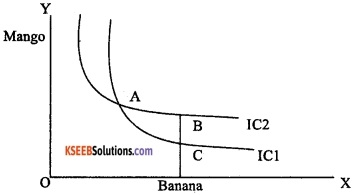In the above diagram, the two indifference curves have intersected with each other. As points A and B lie on IC2, utilities derived ManS° from A and B are same. Similarly, as points A and C lie on the same indifference curve IC1, the utilities are same. From this, it follows that utility from point B and C are same.

But this is clearly an absurd result as on B, the consumer gets a greater number of mangoes with the same quantity of bananas. So the consumer is ‘ better off at point B than at point C. Thus, it is clear that intersecting indifference curves will lead to conflicting results. Thus, two indifference curves cannot intersect each other.

Question 46.
Explain the simultaneous shifts of demand and supply curve in perfect competition with the help of diagrams.
Answer:
The simultaneous shifts can happen in four possible ways:

1. Both supply and demand curves shift rightwards.
2. Both supply and demand curves shift leftwards.
3. Supply curve shifts leftward and demand curve shifts rightward.
4. Supply curve shifts rightward and demand curve shifts leftward.

The simultaneous shifts of demand and supply curve in perfect competition can be represented in the following table: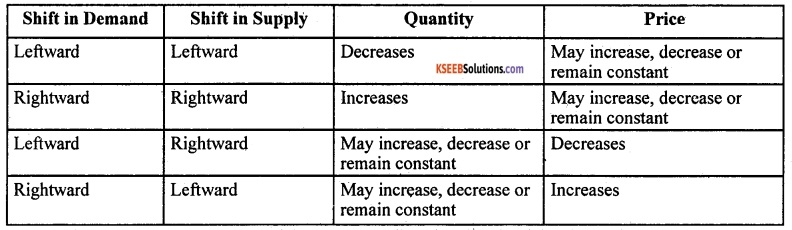In the above table, each row of the table describes the direction in which the equilibrium price and quantity will change for each possible combination of the simultaneous shifts in demand and supply curves. For instance, from the second row of the table, we can notice that due to a rightward shift in both demand and supply curves, the equilibrium quantity increases invariably but the equilibrium price may increase or decrease, or remain constant.
The following diagrams depict the second and third cases of the above table: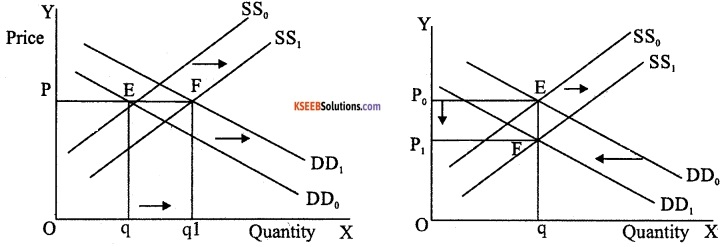In the above diagram (a) initially, the equilibrium is at E where the demand curve DD0 and supply curve SS0 intersect. Here, both supply and demand curves shift rightward where the price remains constant at P but the equilibrium quantity moves from q to q1.

Similarly, in diagram (b), the supply curve shifts rightward and demand curve shifts leftward where the equilibrium quantity remains same but the equilibrium price decreases from P to P1.

Therefore, the rightward shifts in both demand and supply curves leads to increase in the equilibrium quantity and equilibrium price remaining constant. The equilibrium quantity remains same and the price decreases if there is leftward shift in demand curve and a rightward shift in supply curve.Question 47.
Explain how the firms behave in oligopoly.
Answer:
If the market of a particular commodity consists of a few number of sellers, the market structure is termed oligopoly. Given there are a few firms, each firm is relatively large when compared to the size of the market. As a result each firm is in a position to affect the total supply in the market and thus influence the market price.

For example, if a firm decides to double its output, the total supply in the market will increase, causing the price to fall. This fall in price affects the profits of all firms in the industry. Other firms will respond to such a move in order to protect their own profits, by taking fresh decisions regarding how much to produce. Therefore the level of output in the industry, the level of prices and the profits are outcomes of how firms are interacting with each other.

Case-1:
Firms could decide to collude with each other to maximize profits. Here the firms form a Cartel (an association) that acts as a monopoly. The quantity supplied collectively by the industry and the price charged are the same as a single monopoly firm.

Case-2:
The firms could decide to compete with each other. For example, a firm may lower its price a little below the other firms, in order to attract away their customers. Certainly, the other firms would retaliate by doing the same. So the market price keeps falling.

In reality, cooperation of the kind that is needed to ensure a monopoly outcome is often difficult to achieve in the real world. The firms may realize that competing fiercely by continuous price cuts is harmful to their own profits.

Question 48.
Write down some of the limitations of using GDP as an index of welfare of a country.
Answer:
Gross domestic product (GDP) is the sum total of value of goods and services created within the geographical boundary of a country in a particular year. It gets distributed among the people as incomes except retained earnings.

So, we consider that higher level of GDP of a country is an index of greater well being of the people of that country. Welfare of a country means well being of entire population of the country. But there are certain limitations of using GDP as an index of welfare of a country. They are as follows:

1. Distribution of gross domestic product (GDP):
Generally, the rise in GDP will not represent increase in the welfare of the country. If the GDP of the country is rising, the welfare may not rise as a consequence. This is because the rise in gross domestic product may be concentrated in the hands of very few individuals or firms. For the remaining, the income may, in fact, might have decreased. In such a situation, the welfare of the entire country cannot be said to have improved.

2. Non-monetary exchanges:
Some of the activities in a country are not evaluated in terms of money. For instance, the domestic services of housewife are not paid for. The exchanges which take place in the informal sector without the help of money are called barter exchanges. In barter exchanges, goods are directly exchanged against each other.

As money is not used here, these exchanges are not registered as part of economic activity. In India, because of many remote areas, these kinds of exchanges still take place and they are generally not counted in the GDP. Therefore, gross domestic product calculated in the standard manner may not give us a clear indication of welfare of a country.

3. Externalities:
An externality is a cost or benefit conferred upon second or third parties as a result of acts of individual production and consumption. In other words, externalities refer to the benefits or harms, a firm or an individual causes to another for which they are not paid or penalized. These do not have any market in which they can be bought and sold.

But the cost or benefit of an externality cannot be measured in money terms because it is not included in market activities. For example, the pleasure one gets from his neighbour’s garden is an external benefit and external cost is environmental pollution caused by industries. Both are excluded from national income estimates.

4. Leisure and work:
One of the important things that affect the welfare of a society is leisure. But is not included in GDP. For example, longer working hours may make people unhappy because their leisure is reduced. On the contrary, shorter working hours per week may increase leisure and make people happy.

5. Manner of production:
The economic welfare also depends on the manner of production of goods and services. If goods are produced by child labour or by exploitation of workers, then the economic welfare cannot increase.

PART-E

VIII. Answer any two of the following project oriented questions. (5 × 2=10)

Question 49.
Compute the total revenue, marginal revenue and average revenue schedules from the following table when market price of each unit of goods is Rs.10.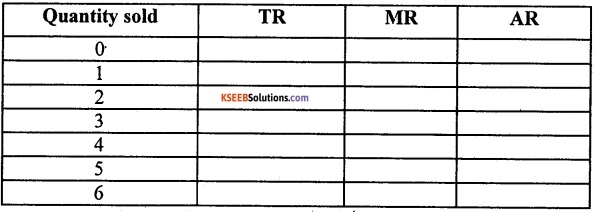Answer:
Hint: For TR multiply price and quantity (P × Q);
MR = TRn – TRn-1 and AR = TR/Q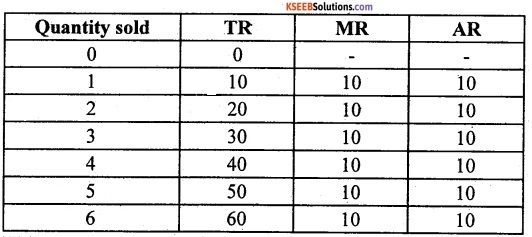Question 50.
Write a note on demonetisation.
Answer:
Demonetisation was a new step taken by the Government of India on 8th November 2016. It was introduced to tackle the problem of corruption, black money, terrorism and circulation of fake currency in the economy. Old currency notes of Rs.500 and Rs. 1000 were no longer legal tender.

New currency notes in denomination of Rs.500 and Rs.2000 were introduced. The public were advised to deposit old currency notes in their bank account till 31st of March 2017 without any declaration and after 31st March 2017 with the RBI with declaration.

In order to avoid a complete Breakdown and scarcity of Cash, government allowed exchange of Rs.4000 old currency notes with new currency restricting to a person per day. Further, till 12th December 2016, old currency notes were acceptable as legal tender at petrol pumps, government hospitals and for payment of government dues like taxes, power bills, etc.

This initiative had both appreciation and criticism. There were long queues outside banks and ATM centres. There was acute shortage of currency notes and had adverse effect on economic activities. But now, normalcy has returned.

The demonetization also has positive effects. It improved tax compliance as a large number of people were bought in the tax ambit. The savings of individual were channelized into the formal financial system. As a result, banks have more resources at their disposal which can be used to provide more loans at low rate of interest.

Demonetisation helps in curbing black money, reducing tax evasion and corruption will decrease. It also helps in tax administration in another way, by shifting transaction out of the cash economy into the formal payment system. Nowadays, households and “firms have started to shift from cash payment to electronic payments.Question 51.
Prepare a budget on monthly income and expenditure of your family.
Answer:
The budget is a financial statement which includes anticipated income and anticipated expenditure. An imaginary monthly income and expenditure of a family is given below:
Budget for the month of January-2019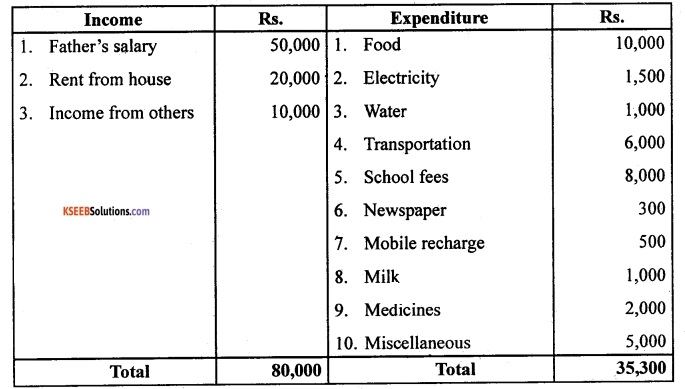This family has a surplus budget as its income is more than expenditure.